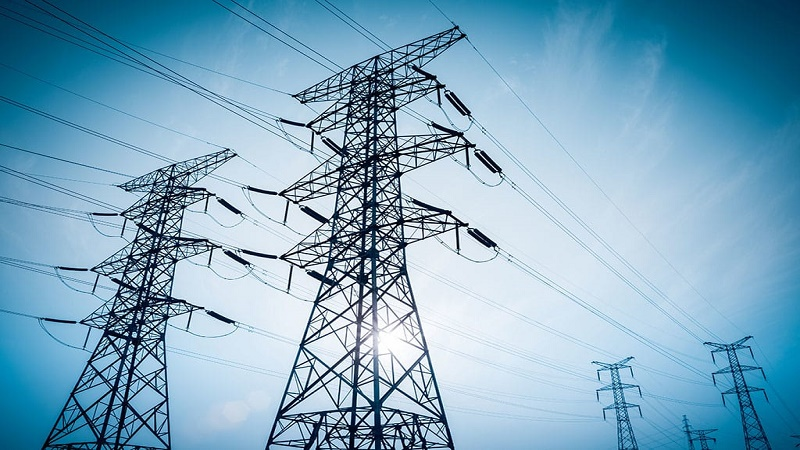Power System Analysis - EE8501, EE6501

Online Study Material, Lecturing Notes, Assignment, Reference, Wiki and important questions and answersPOWER SYSTEM INTRODUCTION

=> Power System Analysis - Introduction
=> Modern Power System (Or) Electric Energy System
=> Analysis For System Planning and Operational Studies
=> Basic Components of a Power System
=> Concept of Real and Reactive Power
=> Modeling of Components For Load Flow Analysis
=> Single Line diagram of an Electrical system
=> Impedance Diagram
=> Reactance Diagram
=> Per Phase and Per Unit Representation
=> Change of Base
=> Formation of Y BUS & Z BUS
=> Solved problems and Examples on Per Unit Analysis
=> Important Short Questions and Answers: Power System Analysis - Introduction

POWER FLOW ANALYSIS

=> Importance of Power Flow Analysis in Planning and Operation of Power Systems
=> Statement of Power Flow Problem
=> Classification of Buses
=> Iterative Solution Using Gauss-Seidel Method - Algorithm
=> Iterative Solution Using Newton-Raphson Method - Algorithm
=> Iterative Solution Using Fast Decoupled Load Flow Method - Algorithm
=> Compare the Gauss Seidel and Newton Raphson Methods of Load Flow Study
=> Important Short Questions and Answers: Power Flow Analysis

SYMMETRICAL or BALANCED FAULT ANALYSIS

=> Importance Short Circuit (Or) For Fault Analysis
=> Basic Assumptions in Fault Analysis of Power Systems
=> Symmetrical Fault
=> Fault Analysis Using Z-Bus Matrix - Algorithm
=> Solved problems: Fault Analysis - Balanced Faults
=> Important Short Questions and Answers: Fault Analysis - Balanced Faults

UNSYMMETRICAL or UNBALANCED FAULT ANALYSIS

=> Introduction to Symmetrical Components
=> Sequence Impedances Sequence Networks
=> Representation of Single Line to Ground, Line to Line and Double Line to Ground Fault Conditions
=> Unbalanced Fault Analysis Problem Formulation
=> Important Short Questions and Answers: Symmetrical Components And Unbalanced Fault Analysis

STABILITY ANALYSIS

=> Classification of Power System Stability - Angle and Voltage Stability
=> Power angle equation and draw the power angle curve
=> Swing equation for a SMIB (Single machine connected to an infinite bus bar) system
=> Critical clearing angle
=> Single Machine Infinite Bus (SMIB) System: Development of Swing Equation
=> Critical clearing angle and critical clearing time in transient stability
=> Important Short Questions Answers: Stability Analysis

EE6501 Power System Analysis - Anna University 2013 Regulation Syllabus - Download Pdf
EE8501 Power System Analysis - Anna University 2017 Regulation Syllabus - Download Pdf